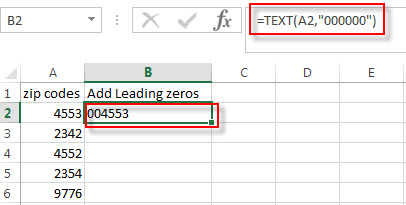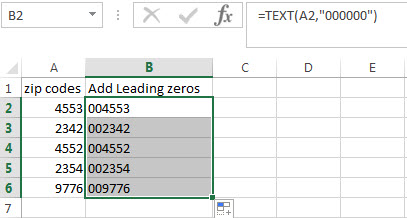# How to insert leading zeros to number or text

This post explains that how to insert or add leading zeros in front of a number or text string in excel. How do add the leading zero to the front of the number using Text function in excel.

If you have a list of zip codes or employee ID that were once text with leading zeros, However when you type a zip code like “021103“ in a cell, then you will find excel will truncate it to “21103” immediately. So how to keep leading zeros in the front of zip code in cell.

Assuming that you want to insert leading zeros  to number in Cell A2, then you can try to use the TEXT function to create an excel formula as follows:

`=TEXT(A2,"000000")`

The number format “000000” in TEXT formula will convert the number to a 6 digit number. If a number is greater than zero, then the number will be displayed, otherwise a zero will be displayed. The last result will be stored as Text format.

1# enter the above formula in the Cell C2, then press enter key2# click AutoFill handle down to all other cells. You will see that all the zip codes in column A are copied to the column C with leading zeros.You can also use the CONCATENATE function to add the specific digit of leading zeros into each number, such as, adding three leading zeros in the front of the zip code, just use the following formula:

`=CONCATENATE("000",A2)`### Related Functions

• Excel Concat function
The excel CONCAT function combines 2 or more strings or ranges together.This is a new function in Excel 2016 and it replaces the CONCATENATE function.The syntax of the CONCAT function is as below:=CONCAT (text1,[text2],…)…
• Excel Text function
The Excel TEXT function converts a numeric value into text string with a specified format. The TEXT function is a build-in function in Microsoft Excel and it is categorized as a Text Function. The syntax of the TEXT function is as below: = TEXT (value, Format code)…

### Related Posts

• How to keep or remove leading zeros
If you want to keep the leading zeros as you type in a cell, you need to change the cell format to Text before you type the zip code number.You can remove or delete leading zeros in front of the cells with excel VBA macro…
• Count Numbers with Leading Zeros
Suppost you have a list of product ID in your worksheet, and the product ID contains the different number of leading zeros in the front of string. how to preserve the leading zeros while counting numbers. And you can use another Excel function named as SUMPRODUCT.…